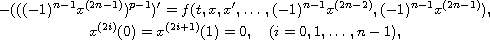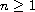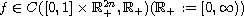Electron. J. Diff. Equ., Vol. 2013 (2013), No. 36, pp. 1-14.

### Positive solutions for a 2nth-order p-Laplacian boundary value problem involving all derivatives Youzheng Ding, Jiafa Xu, Xiaoyan Zhang

Abstract:
In this work, we are mainly concerned with the positive solutions for the 2nth-order p-Laplacian boundary-value problemwhereand. To overcome the difficulty resulting from all derivatives, we first convert the above problem into a boundary value problem for an associated second order integro-ordinary differential equation with p-Laplacian operator. Then, by virtue of the classic fixed point index theory, combined with a priori estimates of positive solutions, we establish some results on the existence and multiplicity of positive solutions for the above problem. Furthermore, our nonlinear term f is allowed to grow superlinearly and sublinearly.

Submitted September 10, 2012. Published January 30, 2013.
Math Subject Classifications: 34B18, 45J05, 47H1.
Key Words: Integro-ordinary differential equation; a priori estimate; index; fixed point; positive solution.

Show me the PDF file (253 KB), TEX file, and other files for this article.Youzheng Ding School of Mathematics, Shandong University Jinan 250100, Shandong, China email: dingyouzheng@139.com Jiafa Xu School of Mathematics, Shandong University Jinan 250100, Shandong, China email: xujiafa292@sina.com Xiaoyan Zhang School of Mathematics, Shandong University Jinan 250100, Shandong, China email: zxysd@sdu.edu.cn